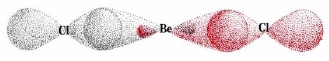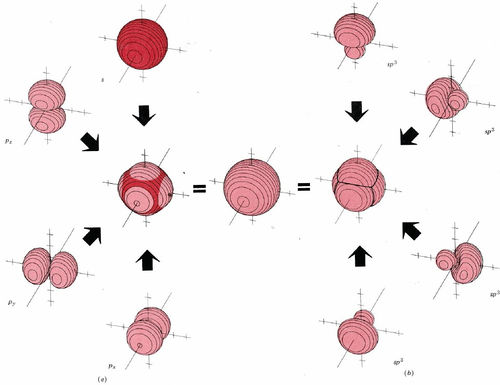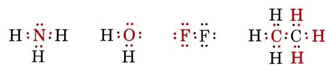# 7.5: Hybrid Orbitals

In the sections on chemical bonding we showed that a covalent bond results from an overlap of atomic orbitals—usually one orbital from each of two bonded atoms. Maximum bond strength is achieved when maximum overlap occurs. When we try to integrate this idea of orbital overlap with VSEPR theory, however, a problem arises. For example, the 2s and 2p atomic orbitals for a C atom are either spherically symmetrical (2s) or dumbbell shaped at angles of 90° to each other (2px2py). The VSEPR theory predicts that the C―Cl bonds in CCl4 are oriented at angles of 109.5° from one another and that all four C―Cl bonds are equivalent. If each C―Cl bond is formed by overlap of Cl orbitals with C 2s and 2p orbitals, it is hard to understand how four equivalent bonds can be formed. It is also difficult to see why the angles between bonds are 109.5° rather than the 90° angle between p orbitals

### sp Hybrid Orbitals

To understand molecular shapes, we first need to consider a simple case, that of beryllium chloride. As seen in Figure 1 from The Shapes of Molecules, VSEPR theory predicts a linear BeCl2 molecule with a Cl—Be—Cl angle of 180°, in agreement with experiments. However, if we associate two valence electron pairs with a beryllium atom and place them in the lowest energy orbitals, we obtain the configuration 2s2p22. (Note that since the electrons must be paired in order to form bonds, they do not obey Hund’s rule.) The electron density distribution of such a configuration is shown in Figure 1a. The 2s cloud is indicated in red, and the 2p cloud in gray.

Figure $$\PageIndex{1}$$: Electron-density distribution for the valence electron configuration 2s22px2. Use the buttons at the bottom (you may need to scroll down) to toggle orbitals off and on. (a) In the top image, color coded to shows s (red) and px (gray) electron densities. (b) Color coded to show the two different sp hybrid orbital electron densities. (Computer generated.)

An important aspect of Figure $$\PageIndex{1}$$ a the fact that the density of electron probability is greater along the x axis than in any other direction. This can be seen more clearly in Figure $$\PageIndex{1}$$ b where the dots have been color coded to indicate one electron pair to the left and one to the right of the nucleus. In this case we should certainly predict that chlorine atoms bonded to the beryllium through these electron pairs would lie on a straight line, the x axis. Each of the two orbitals whose electron densities are shown in Figure $$\PageIndex{1}$$ b is called an sp hybrid. The word hybrid indicates that each orbital is derived from two or more of the atomic orbitals discussed in sections on the Electronic Structure of Atoms, and the designation sp indicates that a single s and a single p orbital contributed to each sp hybrid.

Careful comparison of the s and p electron densities with those of the two sp hybrid orbitals will reveal another important fact. For every dot in Figure $$\PageIndex{1}$$ a, there is a corresponding dot (in the same location) in Figure $$\PageIndex{1}$$ b. That is, the overall electron density (due to the four electrons occupying two orbitals) is exactly the same in both cases. We have not created something new with the two sp hybrids. Rather, we are looking at the same electron density, but we have color coded it to emphasize its concentration along the x axis. In actual fact all electrons are identical—we cannot distinguish one from another experimentally. Labeling one electron cloud as s and another as p is an aid to our thinking, just as color-coding one sp-hybrid electron density red and the other gray is, but it is the overall electron density which determines the experimentally observable molecular geometry. In other words, it does not matter to the molecule whether we think of the total electron cloud as being formed from s and p orbitals or from sp hybrids, but it does matter to us. It is much easier to think of two Be—Cl bonds separated by 180° in terms of sp hybrids than in terms of separate s and p orbitals. By contrast it is much easier to explain the periodic table by using s and p orbitals rather than hybrids. Since both correspond to the same physical reality, we can use whichever approach suits us best.

When beryllium forms a linear molecule such as beryllium chloride, it is not the sp hybrids themselves that form the two bonds but rather an overlap between each of these orbitals and some orbital on each other atom. The situation is shown schematically in Figure $$\PageIndex{2}$$ . As a result of each orbital overlap, there is a concentration of electron density between two nuclei. This pulls the nuclei together and forms a covalent bond.

### sp2 Hybrid Orbitals

Somewhat more complex hybrid orbitals are found in BCl3, where the boron is surrounded by three electron pairs in a trigonal arrangement. If we place these three electron pairs in the valence shell of boron, one 2s and two 2p orbitals (2px and 2py, for example) will be filled, giving the electron configuration 2s22222y. This configuration is illustrated in Figure $$\PageIndex{3}$$ a as a dot-density diagram in which each electron pair is represented in a different color. Immediately below this diagram is another (Figure $$\PageIndex{3}$$ b) which is dot-for-dot the same as the upper diagram but with a different color-coding. The total electron density distribution is the same in both diagrams, but in the bottom diagram the three electron pairs are distributed in a trigonal arrangement.All three electron clouds are identical in shape, and they are oriented at angles of 120° with respect to each other. Again we have a set of hybrid orbitals, but this time they are derived from one s orbital and two p orbitals. Accordingly these orbitals are known as sp2 hybrids.

Because electrons are indistinguishable, and because both the upper and the lower diagrams in Figure $$\PageIndex{3}$$ correspond to an identical total electron density, we are entitled to use either formulation when it suits our purposes. To explain the trigonal geometry of BCl3 and similar molecules, the hybrid picture is obviously more suitable than the s and p picture. The three electron-pair bonds usually formed by boron result from an overlap of each of these three sp2 hybrids with a suitable orbital in each other atom.

Figure $$\PageIndex{3}$$ Electron-density distribution for the valence electron configuration 2s22p2x2p2y. (a) Color coded to show 2s (black), 2px (green), and 2py (blue) electron densities; (b) color coded to show electron densities of three sp2 hybrids at 120° angles. (Computer-generated.)

### sp3 Hybrid Orbitals

In addition to the two hybrids just considered, a third combination of s and p orbitals, called sp3 hybrids, is possible. As the name suggests, sp3 hybrids are obtained by combining an s orbital with three p orbitals (px, py and pz). Suppose we have two s electrons, two px electrons, two py electrons, and two pz electrons, as shown by the boundary-surface diagrams in Figure $$\PageIndex{3}$$. When these are all arranged around the nucleus, the total electron cloud is essentially spherical. The boundary-surface diagrams in Figure $$\PageIndex{4}$$ show a slightly “bumpy” surface, but we must remember that electron clouds are fuzzy and do not stop suddenly at the boundary surface shown. When this fuzziness is taken into account, the four atomic orbitals blend into each other perfectly to form an exactly spherical shape. This blending of s and p orbitals is much the same as that discussed in the previous cases of sp and sp2 hybrids. It can be clearly seen in two dimensions in Figure $$\PageIndex{1}$$ or Figure $$\PageIndex{3}$$ a. The total electron probability cloud in Figure $$\PageIndex{4}$$ can be subdivided in a different way―into four equivalent sp3 hybrid orbitals, each occupied by two electrons. Each of these four hybrid orbitals has a similar appearance to each of the sp and sp2 hybrids encountered previously, but the sp3 hybrids are arranged tetrahedrally around the nucleus. The four sp3 orbitals, each occupied by two electrons, also appear bumpy in a boundary-surface diagram, but when the fuzziness of the electron clouds is taken into account, the result is a spherical electron cloud equivalent in every way to an ns2np6 configuration. We are thus equally entitled to look at an octet of electrons in terms of four sp3 hybrids, each doubly occupied, or in terms of one s and three p orbitals, each doubly occupied. Certainly, nature cannot tell the difference!

According to the VSEPR theory, if an atom has an octet of electrons in its valence shell, these electrons are arranged tetrahedrally in pairs around it. Since sp3 hybrids also correspond to a tetrahedral geometry, they are the obvious choice for a wave-mechanical description of the octets we find in Lewis structures. Consider, for example, a molecule of methane, CH4, whose Lewis structure isHere the eight electrons of the octet can be thought of as occupying four orbitals, each formed by the overlap of an sp3 hybrid centered on the C atom and a 1s orbital belonging to an H atom. A schematic diagram of this bonding scheme is shown in Figure $$\PageIndex{5}$$ .Also shown in this figure are four other simple molecules whose Lewis structures areIn each case the octets in the Lewis structure can be translated into sp3 hybrids. These sp3 hybrids can either overlap with the 1s orbitals of H or with sp3 hybrids in other atoms. Alternatively they can form lone pairs, in which case no bond is formed and no overlap is necessary.

Example $$\PageIndex{1}$$ : Hybrid Orbitals

Suggest which hybrid orbitals should be used to describe the bonding of the central atom in the following molecules:

1. BeF2,
2. PbF2
3. SbCl3
4. InCl3

Solution

In making decisions on which hybrids need to be used, we must first decide on the shape of the molecule from VSEPR theory. This in turn is best derived from a Lewis structure. The Lewis structures of these four molecules are1. In BeF2 there are only two electron pairs in the valence shell of Be. These must be arranged linearly according to VSEPR theory thus necessitating two sp hybrid orbitals.
2. In PbF2 there are three electron pairs in the valence shell of Pb. According to VSEPR theory these will be arranged trigonally and will thus utilize sp2 hybrids.
3. In SbCl3 there is an octet of electrons—four pairs arranged tetrahedrally. Accordingly sp3 hybrids are needed to describe the bonding.
4. The atom of In has only three pairs of electrons in its valence shell. As in case b, this produces a trigonal arrangement of electron pairs and is described by sp2 hybridization.

### Other Hybrid Orbitals

Hybrid orbitals can also be used to describe those shapes which occur when there is more than an octet of electrons in an atom’s valence shell. The combination of a d orbital with an s orbital and three p orbitals yields a set of five dsp3 hybrids. These dsp3 hybrids are directed toward the corners of a trigonal bipyramid. An octahedral arrangement is possible if one more d orbital is included. Then d2sp3 hybrids, all at 90° to one another, are formed. Note that as soon as we get beyond an octet (whose eight electrons fill all the s and p orbitals), the inclusion of d orbitals is mandatory. This can only occur for atoms in the third row of the periodic table and below. Thus bonding in PCl5 involves dsp3 hybrid orbitals which include a P 3d orbital. Bonding in SF6 uses two S 3d orbitals in d2sp3 hybrids.